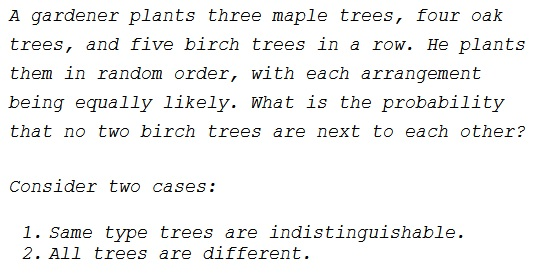# Planting Trees in a Row

### Problem### Solution 1, Part 1

Let's do it in general, with $m$ maple trees, $o$ oak trees, and $b$ birch trees.

There is a total $T$ of arrangements of the three kinds of trees: $\displaystyle T=\frac{(m+o+b)!}{m!\,o!\,b!};$ $\displaystyle M={m+o\choose o}=\frac{(m+o)!}{m!\,o!}$ ways to arrange maple and oak trees; and $\displaystyle N={m+o+1\choose b}=\frac{(m+o+1)!}{(m+o+1-b)!\,b!}$ ways to place $b$ beach trees so that no two are adjacent. The probability we are interested in is

\displaystyle \begin{align} P&=\frac{M\cdot N}{T}=\frac{(m+o)!}{m!\,o!}\cdot\frac{(m+o+1)!}{(m+o+1-b)!\,b!}\cdot\frac{m!\,o!\,b!}{(m+o+b)!}\\ &=\frac{(m+o)!\,(m+o+1)!}{(m+o+1-b)!\,(m+o+b)!}=\frac{(m+o+1)!}{(m+o+1-b)!\,b!}\cdot\frac{(m+o)!\,b!}{(m+o+b)!}\\ &=\frac{m+o+1\choose b}}{m+o+b\choose b}}. \end{align

### Solution 1, Part 2

There is a total $T=(m+o+b)!$ of ways to plant the trees. Maples and oaks can be planted in $M=(m+o)!$ ways distinct ways, creating $m+o+1$ places for single birch trees. These can be planted there in $\displaystyle N=\frac{(m+o+1)!}{(m+o+1-b)!}$ unique ways. The probability we are after is

\displaystyle \begin{align} P&=\frac{M\cdot N}{T}=\frac{(m+o)!\cdot (m+o+1)!}{(m+o+1-b)!}\cdot\frac{1}{(m+o+b)!}\\ &=\frac{(m+o)!\cdot (m+o+1)!\cdot b!}{(m+o+1-b)!}\cdot\frac{1}{(m+o+b)!\cdot b!}\\ &=\frac{(m+o+1)!}{(m+o+1-b)!\,b!}\cdot\frac{(m+o)!\,b!}{(m+o+b)!}\\ &=\frac{m+o+1\choose b}}{m+o+b\choose b}}. \end{align

### Solution 2

#### Distinguishable case

First order the maple and the oak trees ($7!$ ways). Plant the birch trees in $5$ of the possible $8$ locations (either side of the planted trees or in the middle of two planted trees) for each birch tree ($P(8,5)$ ways). Without restrictions, there are $12!$ ways to plant. Thus, the desired probablility is

$\displaystyle P_d=\frac{7!\cdot P(8,5)}{12!}=\frac{7}{99}.$

#### Indistinguishable case

This is same as the distinguishable case other than the fact that the number of ways with and without the constraint both get scaled down by $3!4!5!$- the number of permutations among the trees of the same kind. As a result, the ratio does not change and the probability is unchanged.

### Remark

Perhaps surprisingly, the two cases produce exactly the same result. No less surprisingly, the answer is a fraction of two binomial coefficients which are the staples of problems dealing with indistinguishable objects. As a matter of fact the distinction between maples and oaks is a red herring, the only quantity that matters is their total amount. However, the distinction between maples and oaks on one hand and birch trees on the other has to be accounted for in the solution.

So there is the total of ${m+o+b\choose b}$ ways select "birches" among the whole bunch of the trees. The remaining trees create $m+o+1$ spaces to be filled individually by those selected.

The answer in both cases is $\displaystyle \frac{7}{99}.$ Finding it with the specific amounts of the tree makes that fact more surprising than it deserves. The general (algebraic) solution sheds some light on the commonality of the two cases.

### Acknowledgment

This page is based on a problem from Section 10 of Ross Honsberger's Mathematical Delights (MAA, 2004). According to the book, this is problem #11 from the 1984 AIME Contests.

Solution 2 is by Amit Itagi.